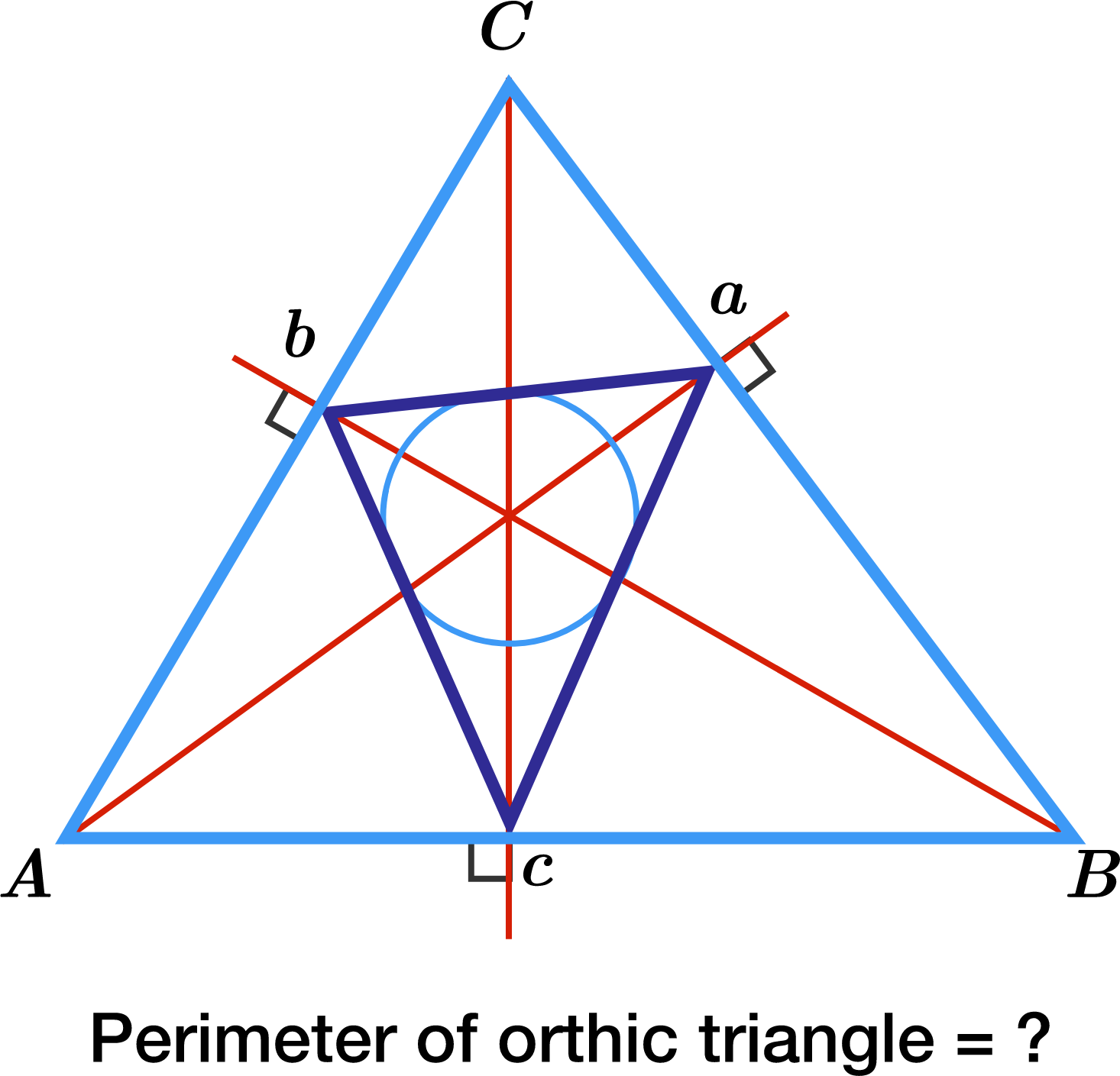# Relationship Between Orthic Triangle And Original Triangle?

Geometry Level 5Let $ABC$ be a triangle with sides 13, 14 and 15. If the perimeter of its orthic triangle can be represented as $\dfrac {p}{q}$, where $p$ and $q$ are coprime positive integers, what is the value of $p+q$?

×